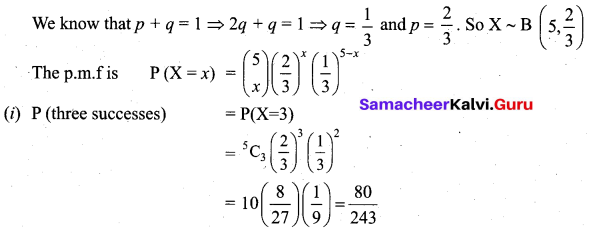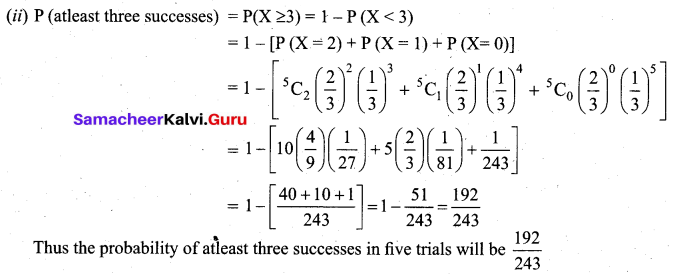# Samacheer Kalvi 12th Business Maths Solutions Chapter 7 Probability Distributions Ex 7.1

Students can download 12th Business Maths Chapter 7 Probability Distributions Ex 7.1 Questions and Answers, Samacheer Kalvi 12th Business Maths Book Solutions Guide Pdf helps you to revise the complete Tamilnadu State Board New Syllabus and score more marks in your examinations.

## Tamilnadu Samacheer Kalvi 12th Business Maths Solutions Chapter 7 Probability Distributions Ex 7.1

Question 1.
Define Binomial distribution.
Solution:
A random variable X is said to follow a binomial distribution with parameter ‘n’ and ‘p’ if it assumes only non-negative value and its probability mass function is given by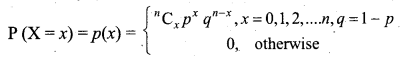Question 2.
Define Bernoulli trials.
Solution:
A random experiment whose outcomes are of two types namely success S and failure F, occurring with probabilities p and q respectively, is called a Bernoulli trial.
Some examples of Bernoulli trials are:
(i) Tossing of a coin (head or tail)
(ii) Throwing of a die (getting even or odd number)Question 3.
Derive the mean and variance of the binomial distribution.
Solution:
Let X be a random variable with the Binomial distribution.
The probability function of X is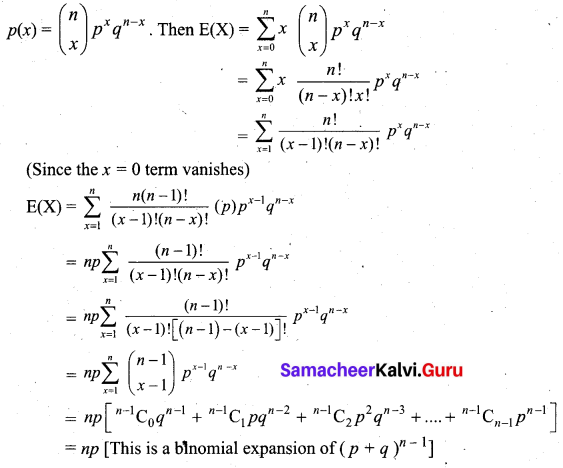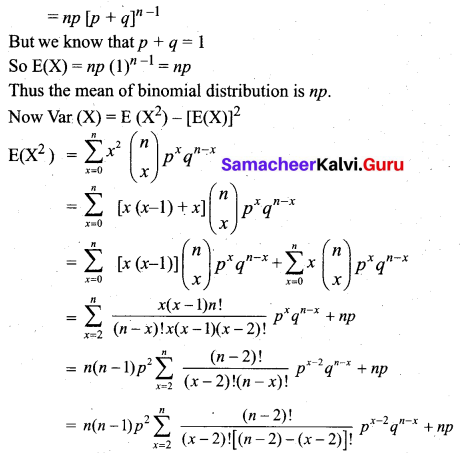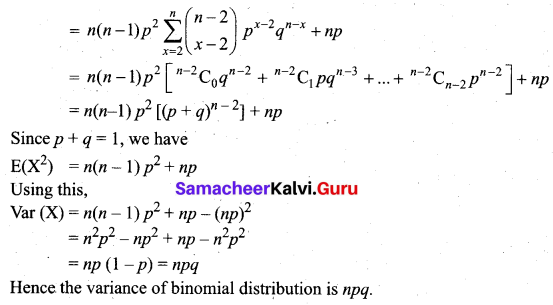Question 4.
Write down the conditions for which the binomial distribution can be used.
Solution:
The Binomial distribution can be used under the following conditions:

1. The number of trials ‘n finite
2. The trials are independent of each other.
3. The probability of success ‘p’ is constant for each trial.
4. In every trial there are only two possible outcomes – success or failure.Question 5.
Mention the properties of the binomial distribution.
Solution:
Property 1:
The binomial distribution is symmetrical when the probability of success ‘p’ is 0.5 (or) when a number of trials ‘n’ is very large. In other words, if p = q = 1/2, the distribution is symmetric about the median. If p ≠ q, then it is skewed distribution, (p < 0.5 → positively skewed, p > 0.5 → negatively skewed)

Property 2:
The variance is less than mean (i,e,) npq < np

Question 6.
If 5% of the items produced turn out to be defective, then find out the probability that out of 10 items selected at random there are
(i) exactly three defectives
(ii) at least two defectives
(iii) exactly 4 defectives
(iv) find the mean and variance
Solution:
Let p be the probability of a defective item.
Given that, p = 5% = $$\frac{5}{100}$$ = 0.05
So q = 1 – p = 1 – 0.05 = 0.95. Also n = 10.
Let X be the random variable which follows the binomial distribution. Then X ~ B (10, 0.05)
(i) P(exactly three defectives) = P(X = 3)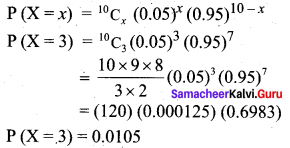(ii) P(atleast two defectives) = P (X ≥ 2) = 1 – P (X < 2)
= 1 – [P(X = 1) + P(X = 0)]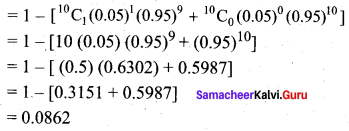(iii) P(exactly 4 defectives) = P(X = 4)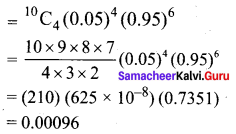(iv) We know that mean = np = (10) (0.05) = 0.5
Variance = npq = (10) (0.05) (0.95) = 0.475Question 7.
In a particular university, 40% of the students are having newspaper reading habits. Nine university students are selected to find their views on reading habits. Find the probability that
(i) none of those selected has a newspaper reading habit
(ii) all those selected have newspaper reading habit
(iii) at least two-third have newspaper reading habits.
Solution:
Let X be the binomial random variable which denotes the number of students having newspaper reading habit.
It is given that 40% of students have reading habit.
p = $$\frac{40}{100}$$ = 0.4 and q = 1 – 0.4 = 0.6
(i) P(none of selected have newspaper reading habit) = P(X = 0)
Now X ~ B (9, 0.4)
The p.m.f is given by P (X = x) = p (x) = $$^{9} \mathrm{C}_{x}(0.4)^{x}(0.6)^{9-x}$$
P(X = 0) = $$^{9} \mathrm{C}_{0}(0.4)^{0}(0.6)^{9}$$ = (0.6)9 = 0.01008 (using calculator)
(ii) P (all selected have newspaper reading habit)
= P (X = 9)
= $$^{9} \mathrm{C}_{9}(0.4)^{9}(0.6)^{0}$$
= (0.4)9
= 0.000262 (using calculator)
(iii) P (at least two third have newspaper reading habit) = P (X ≥ 6)
{9 students are selected. Two third of them means $$\frac{2}{3}$$ (9) = 6}
Now P (X ≥ 6) = P (X = 6) + P (X = 7) + P (X = 8) + P (X = 9)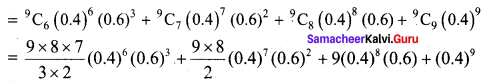= (84) (0.004096) (0.216) + 36 (0.0016384) (0.36) + 9 (0.00065536) (0.6) + 0.000262
= 0.074318 + 0.021234 + 0.003539 + 0.000262
= 0.099353

Question 8.
In a family of 3 children, what is the probability that there will be exactly 2 girls?
Solution:
Let X denote the binomial variable which denotes the number of girls.
Given that n = 3 and p = q = $$\frac {1}{2}$$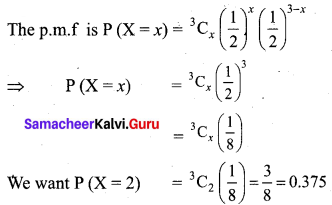Hence the probability that there will be exactly 2 girls is. 0.375.Question 9.
Defects in yarn manufactured by a local mill can be approximated by a distribution with a mean of 1.2 defects for every 6 meters of length. If lengths of 6 metres are to be inspected, find the probability of fewer than 2 defects.
Solution:
Given mean np = 1.2 and n = 6
p = $$\frac{1.2}{6}$$ = 0.2, q = 1 – 0.2 = 0.8
Let X be a binomial variable denoting the number of defects, (i.e,) X ~ B (6, 0.2)
p.m.f is given by P (X = x) = $$^{6} \mathrm{C}_{x}(0.2)^{x}(0.8)^{6-x}$$
We want P(X < 2) = P(X = 0) + P (X = 1)
= $$^{6} \mathrm{C}_{0}(0.2)^{0}(0.8)^{6}+^{6} \mathrm{C}_{1}(0.2)^{1}(0.8)^{5}$$
= (0.8)6 + 6 (0.2) (0.8)5
= 0.262144 + 0.393216
= 0.65536
Thus if lengths of 6 metres are to be inspected, the probability of less than 2 defects is 0.65536.

Question 10.
If 18% of the bolts produced by a machine are defective, determine the probability that out of the 4 bolts chosen at random
(i) exactly one will be defective
(ii) none will be defective
(iii) at most 2 will be defective
Solution:
n = 4
probability of defective bolts p = 18/100
9 = 1 – p = 1.018 = 0.82
The binoial distribution p(x) = 4Cx(0.18)x(0.82)4-x
= 4C1(0.18)1(0.82)4-1
= 4 × 0.18 × (0.82)³
= 0.72 × 0.551368
= 0.39698496
p(X = 1)= 0.3969 approximately

(ii) p(none will be defective)p(x = 0)
= 4C0(0.18)°(0.82)4-0
= (1)(1)(0.45212176)
p(x = 0) = 0.45212

(iii) p(almost 2 will be defective) = p(x ≤ 2)
= p(x = 0) + (p(x = 1) + p(x = 2)
= 4C0(0.18)°(0.82)4-0 + 4C1(0.18)1(0.82)4-1 + 4C2 (0.18)² (0.82)4-2
= (0.82)4 + 4 × (0.18) × (0.82)³ + $$\frac { 4×3 }{1×2}$$ × (0.18)² (0.82)²
= 0.45212176 + (0.72 × 0.551368) + (6 × 0.0324 × 0.6724)
= 0.45212176 + 0.39698496 + 013071456
= 0.97982128
= 0.9798Question 11.
If the probability of success is 0.09, how many trials are needed to have a probability of at least one success as 1/3 or more?
Solution:
Given p = 0.09 (success)
q = 0.91 (failure)
We have to find number of trials ‘n.’
According to the problem,
P(X ≥ 1 ) > $$\frac{1}{3}$$
(We must have atleast one success)
1 – P(X < 1) > $$\frac{1}{3}$$
1 – P(X = 0) > $$\frac{1}{3}$$
(or) P(X = 0) < $$\frac{2}{3}$$
Using p.m.f, we have,
$$^{n} \mathrm{C}_{0}(0.09)^{0}(0.91)^{n}<\frac{2}{3}$$
(0.91)n < $$\frac{2}{3}$$
we can use log tables to calculate (or) by trial method try for n = 1, 2,…… using calculator.
We observe that (0.91)5 < $$\frac{2}{3}$$. Thus we need minimum 5 trial or more.

Question 12.
Among 28 professors of a certain department, 18 drive foreign cars and 10 drive locally made cars. If 5 of these professors are selected at random, what is the probability that at least 3 of them drive foreign cars?
Solution:
Here n = 5, p = $$\frac{18}{28}=\frac{9}{14}$$, q = $$\frac{10}{28}=\frac{5}{14}$$
(i.e.) the probability of professors driving foreign cars p = $$\frac{9}{14}$$, and those who drive local cars q = $$\frac{5}{14}$$.
Let X be the Binomial random variable denoting persons who drive foreign cars.
Then the p.m.f of X is given by P (X = x) = $$^{5} \mathrm{C}_{x}\left(\frac{9}{14}\right)^{x}\left(\frac{5}{14}\right)^{5-x}$$
We want P (X ≥ 3) = P (X = 3) + P (X = 4) + P (X = 5)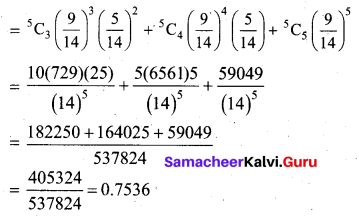Question 13.
Out of 750 families with 4 children each, how many families would be expected to have
(i) at least one boy
(ii) at most 2 girls
(iii) and children of both sexes?
Assume equal probabilities for boys and girls.
Solution:
Given that 750 families are considered each with 4 children. We will find the probabilities for one particular family and then multiply by 750.
In other words, n = 4, p = q = $$\frac{1}{2}$$ (since boy and girl child have equal probability).
Let X denote the binomial random variable which denotes the number of boys in the family.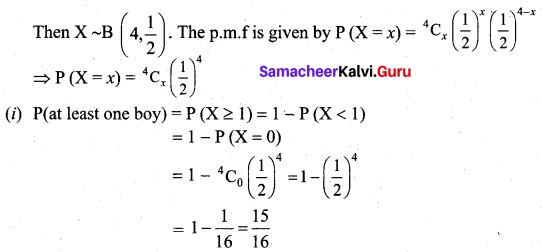So out of 750 families the number of families would be expected to have atleast one boy is $$\frac{15}{16}$$ × 750 = 703
(ii) P(atmost 2 girls) = P(2G, 2B) + P(1G, 3B) + P(0G, 4B)
= P(X = 2) + P(X = 3) + P(X = 4)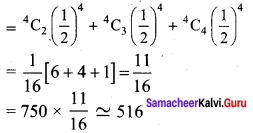Thus out of 750 families, 516 families would be expected to have atmost 2 girls.
(iii) P(children of both sexes) = P(both boys and girls)
Out of 4 children the sample space is given by {BGGG, BBGG, BBBG}and each case in any order.
So we require P(1B, 3G) + P(2B, 2G) + P(3B, 1G)
(i.e,) P(X = 1) + P(X = 2) + P(X = 3)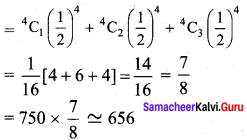Thus out of 750 families, 656 families would be expected to have children of both sexes.

Question 14.
Forty percent of business travellers carry a laptop. In a sample of 15 business travelers
(i) what is the probability that 3 will have a laptop?
(ii) what is the probability that 12 of the travelers will not have a laptop?
(iii) what is the probability that atleast three of the travelers have a laptop?
Solution:
Let X be the binomial variables which denotes the number of business travellers having a laptop.
Given that n = 15 and P = 40% = 0.4. So q = 1 – 0.4 = 0.6. Thus X ~ B (15, 0.4).
The p.m.f of X is given by P (X = x) = $$^{15} \mathrm{C}_{x}(0.4)^{x}(0.6)^{15-x}$$
(i) P(3 travellers will have a laptop) = P (X = 3)Note: The calculation can be done by method of logarithms also.
P(X = 3) = 455 (0.064) (0.002177) = 0.0634
(ii) P(12 of the travellers will not have a laptop)
= P(15 – 12 = 3 will have a laptop)
= P(X = 3) = 0.0634 (from the previous subdivision)
(iii) P(atleast three of the travellers have a laptop)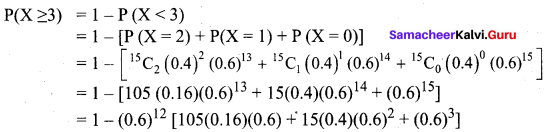Using (0.6)12 = 0.002177 from the previous subdivision, we have
= 1 – (0.002177) [10.08 + 2.16 + 0.216]
= 1 – (0.002177) (12.456)
= 1 – 0.02712
= 0.9729Question 15.
A pair of dice is thrown 4 times. If getting a doublet is considered a success, find the probability of 2 successes.
Solution:
Let p be the probability of getting a doublet, (i.e,) probability of success. When we throw a pair of dice there are 36 possibilities. The number of doublets is 6 [(1, 1) (2, 2), (3, 3) (4, 4) (5, 5) (6, 6)].
So p = $$\frac{6}{36}=\frac{1}{6}$$
q = 1 – $$\frac{1}{6}$$ = $$\frac{5}{6}$$
Let X be the random variable denoting the number of doublet in 4 throws.
Then X ~ B (4, $$\frac{1}{6}[/latex)]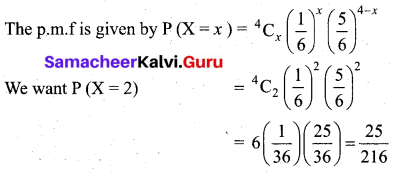Hence the probability of 2 successes is [latex]\frac{25}{216}$$

Question 16.
The mean of a binomial distribution is 5 and the standard deviation is 2. Determine the distribution.
Solution:
Given mean = 5 and standard deviation = 2
(i.e,) np = 5 and √npq = 2 ⇒ npq = 4
5q = 4 ⇒ q = $$\frac{4}{5}$$, p = 1 – $$\frac{4}{5}$$ = $$\frac{1}{5}$$
Again np = 5 gives $$\frac{n}{5}$$ = 5 ⇒ n = 25
So the p.m.f of the distribution is given by P (X = x) = $$\left(\begin{array}{c} 25 \\ x \end{array}\right)\left(\frac{1}{5}\right)^{x}\left(\frac{4}{5}\right)^{25-x}$$Question 17.
Determine the binomial distribution for which the mean is 4 and variance 3. Also find P(X = 15)
Solution:
Given mean = 4 and variance is 3.
(i.e,) np = 4 and npq = 3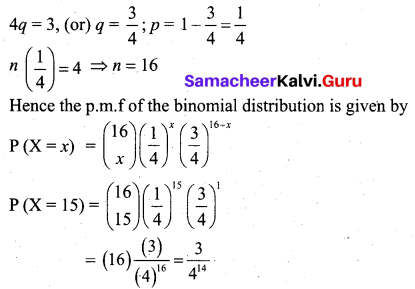Question 18.
Assume that a drug causes a serious side effect at a rate of three patients per one hundred. What is the probability that at least one person will have side effects in a random sample of ten patients taking the drug?
Solution:
According to the problem, n = 10, p = $$\frac{3}{100}$$ = 0.03 where p is the probability that a drug causes side effect. Now X ~ B (10, 0.03). The p.m.f is given by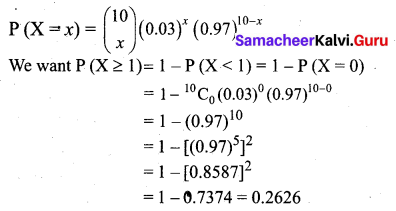Thus the probability that at least one person will have side effects is 0.2626.Question 19.
Consider five mice from the same litter, all suffering from Vitamin A deficiency. They are fed a certain dose of carrots. The positive reaction means recovery from the disease. Assume that the probability of recovery is 0.73. What is the probability that at least 3 of the 5 mice recover?
Solution:
Given n = 5 and the probability of recovery p = 0.73.
So q = 1 – 0.73 = 0.27. X ~ B (5, 0.73).
The p.m.f of X is given by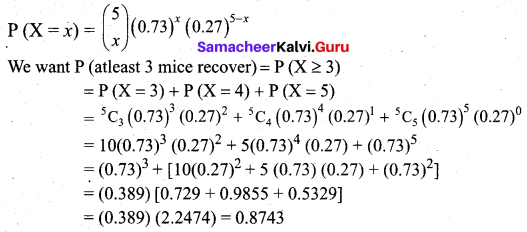Thus the probability that at least 3 of the 5 mice recover is 0.8743.

Question 20.
An experiment succeeds twice as often as it fails, what is the probability that in the next five trials there will be
(i) three successes and
(ii) at least three successes.
Solution:
Given a number of trials n = 5.
Let P be the probability of success and q be the probability of failure. It is given that p = 2q.